﻿

### The submanifolds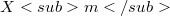$Xm$ of the manifold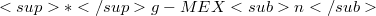$*g-MEXn$. II. Fundamental equations on$Xm$ of$*g-MEXn$

#### Abstract

In our previous paper , we studied the induced connection of the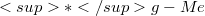$*g-Me$-connection on a submanifold$Xm$ embedded in a manifold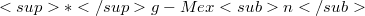$*g-Mexn$ together with the generalized coefficients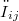$ω_{ij}$ of the second fundamental form of$Xm$, with emphasis on the proof of a necessary and sufficient condition for the induced connection of$Xm$ in$*g-MEXn$ to be a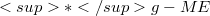$*g-ME$-connection. This paper is a direct continuation of . In this paper, we derive the generalized fundamental equations on$Xm$ of$*g-MEXn$, such as the generalized Gauss formulae, the generalized Weingarten equations, and the Gauss-Codazzi equations. Furthermore, we also present surveyable tensorial representations of curvature tensors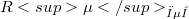$R\mu_{ω\muλ}$ of$*g-MEXn$ and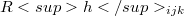$Rh_{ijk}$ of$Xm$.

DOI Code: 10.1285/i15900932v18n2p227

Full Text: PDF